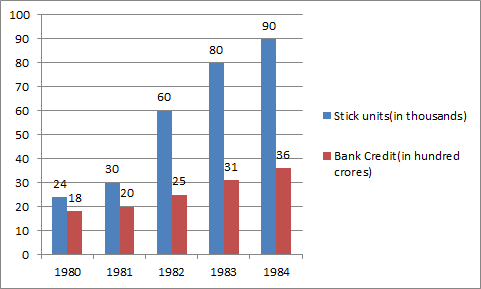# Numerical Reasoning - Test 5

Instructions:

Study the graph carefully and answer the questions1-5 given below1:

What was the percentage increase in number of stick units from 1981 to 1983?

 A. 50 B. 150 C. 25 D. None of these Answer Report Discuss Option: D Explanation : Number of increase in 1981 to 1983 = 80-30 = 50 % = 50/30 * 100 = 166.66% Click on Discuss to view users comments. Write your comments here:
2:

What was the difference in bank credit between 1981 and 1982?

 A. Rs. 5 crore B. Rs. 50 crore C. Rs. 500 crore D. Rs. 50 lac Answer Report Discuss Option: C Explanation : Required difference = (25-20) hundred crore = 5 * 100 crore = 500 crore Click on Discuss to view users comments. Write your comments here:
3:

If the bank credit is reduced to half in the year 1981, what will be the approximate bank credit per stick unit?

 A. Rs. 3 crore B. Rs. 3 lac C. Rs. 30 lac D. Rs. 4 lac Answer Report Discuss Option: B Explanation : Required bank credit per sick unit = Rs. 10 * 100 crore/ 30 * 1000 =Rs. 10 * 1000000000/ 30 * 1000 =Rs. 3.33 Lakhs =Rs. 3 lakhs Click on Discuss to view users comments. Write your comments here:
4:

In which year was the bank credit per stick unit the maximum?

 A. 1980 B. 1981 C. 1982 D. 1983 Answer Report Discuss Option: A Explanation : 1980 = 18 * 100 Crore / 24 * 1000 = Rs. 7.5 lakhs 1981 = 20 * 100 Crore / 30 * 1000 = Rs. 6.6 lakhs 1982 = 25 * 100 Crore / 60 * 1000 = Rs. 4.16 lakhs 1983 = 31 * 100 Crore / 80 * 1000 = Rs. 3.87 lakhs 1984 = 36 * 100 Crore / 90 * 1000 = Rs. 4 lakhs Click on Discuss to view users comments. Write your comments here:
5:

In 1983 what was the total bank credit per stick unit approximately?

 A. Rs. 32 crore B. Rs. 71 crore C. Rs. 71 lac D. Rs. 4 lac Answer Report Discuss Option: D Explanation : Click on Discuss to view users comments. kirti said: (12:55pm on Thursday 1st December 2016) how answer will come 4 lac? Write your comments here:

## Suggest an improvement

1. Take a free Numerical test before your next interview.
2. Take a numeracy psychometric test designed especially for CES and USB
3. Here you can practice questions for ceb numerical reasoning test.
4. These questions can be used for investment banking numerical test .
5. Here you will find questions answers for numeracy psychometric test.
6. This section provides you free online psychometric test.
7. These questions can be used for numerical assessment